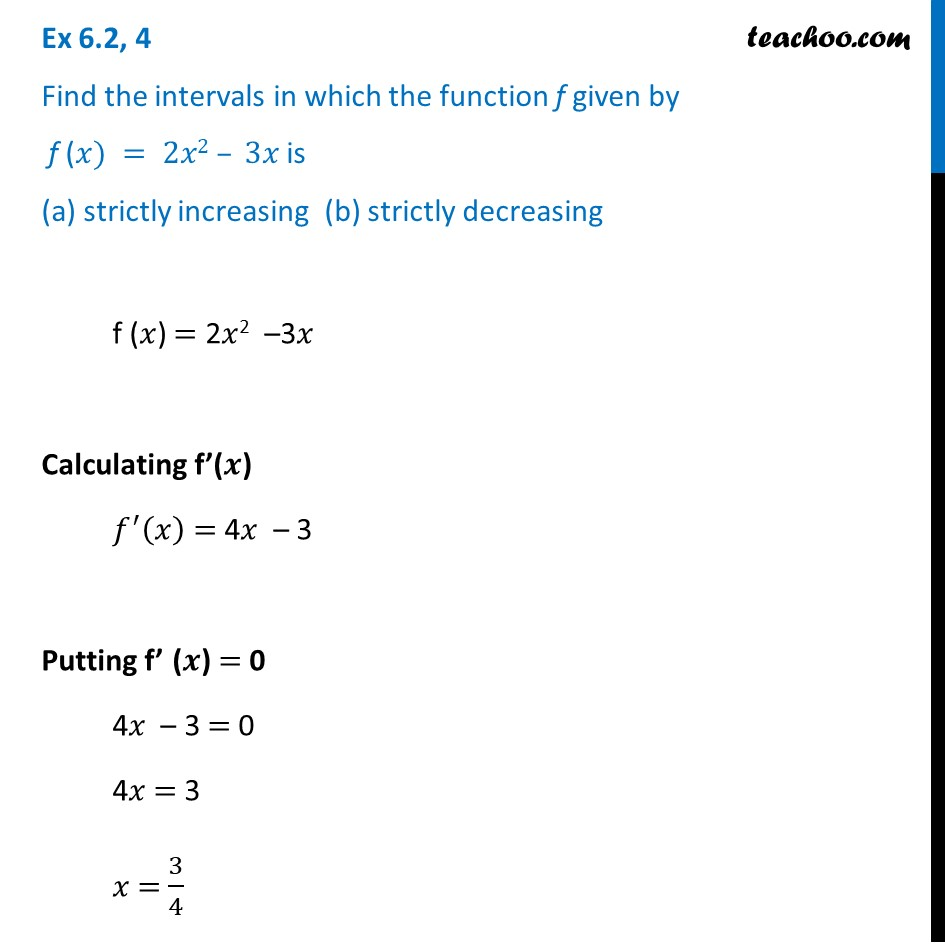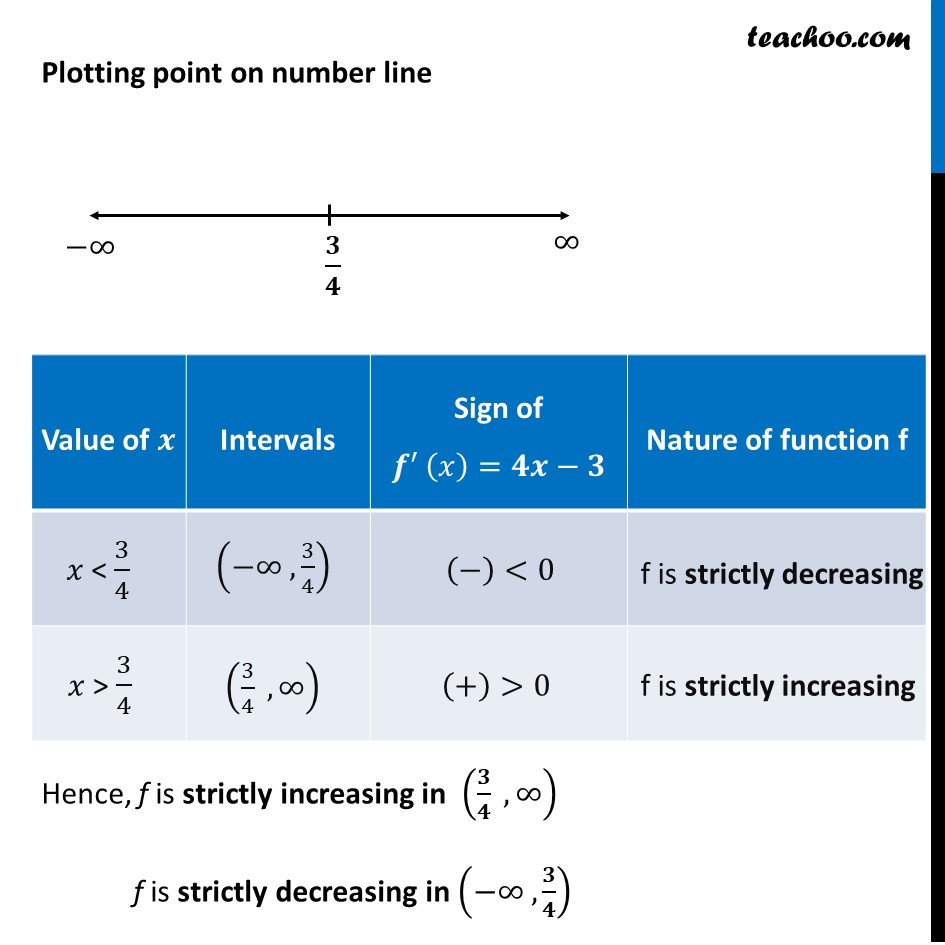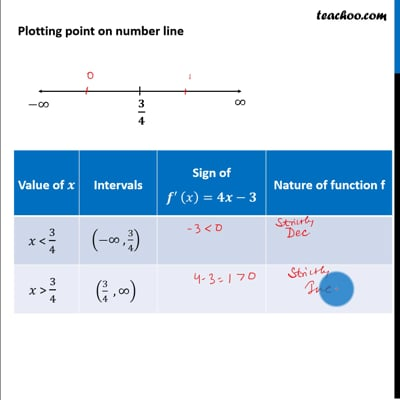Ex 6.2

Chapter 6 Class 12 Application of Derivatives
Serial order wiseThis video is only available for Teachoo black users

Learn in your speed, with individual attention - Teachoo Maths 1-on-1 Class

### Transcript

Ex 6.2, 4 Find the intervals in which the function f given by f (𝑥) = 2𝑥2 – 3𝑥 is (a) strictly increasing (b) strictly decreasing f (𝑥) = 2𝑥2 –3𝑥 Calculating f’(𝒙) 𝑓^′ (𝑥) = 4𝑥 – 3 Putting f’ (𝒙) = 0 4𝑥 – 3 = 0 4𝑥 = 3 𝑥 = 3/4 Plotting point on number line Hence, f is strictly increasing in (𝟑/𝟒 , ∞) f is strictly decreasing in (−∞ ,𝟑/𝟒)